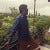# BackPropagation through LSTM: A differential approach

Source: Deep Learning on Medium

LSTM’s are very powerful but they are confusing if you are a beginner. To understand things internally, we need to understand the backpropagation.

So, let’s dive in and try to understand how things work…

For Simplicity, let’s ignore the non-linearities.

### Prerequisites:

This is a slightly advanced topic which requires knowledge of

1. working of LSTM
2. Multi path derivative and partial differentiation
3. Chain Rule and Understanding of Backpropagation.

All the necessary references are mentioned at the end. I strongly recommend going through it if you are new to this.

### Forward Pass:

LSTM consists of cell state(St) and various gates. Cell state is one core component of LSTM and it holds the information that is has learned over time.

LSTM has mainly three gates

1. Keep Gate: It determines what information to keep from the previous state and what to erase.
2. Input Gate: Adds the new information form present input to cell state. Cell state gets updated.
3. Output Gate: Produces the final output vector.

Let’s write down equations for a forward pass. Ignoring the non-linearities i.e all bias terms are set to zero.

During forward pass, we concatenate input x with previous state output i.e [x,h(t-1)] and fed it to LSTM. So, we can write

Z = W x I

where,

I = concatenated input vector

W = weight matrix of input weights and hidden state weights

### Backword Pass:

During backward pass, we calculate local gradients at each step. Gradients are accumulated before being backpropagated the previous time steps.

We write gradient of dl/dh ==> δh. Since this helps us to have the concise notation and avoids repetition of dl every time.

One important point is that gradients are always w.r.t to the final output.

At State, gradient comes from two sources to the top summation node at St. Both paths are mentioned in the above figure.

The gradient at state evaluates to…

Which can be written as

We can write these gradients in terms of input to gates i.e

(W* X + U * h)

as shown in forward pass equations

### Weight Updation:

The aim all the above equations is to find the weights that minimizes loss.

So , the most important question is how to updates weight. We know,

Z = W x I

We can obtain δZ from above equations.

Our aim is to get δh and δW.

δh ==> Useful to back propagate to previous time steps.

δW ==> Useful for weight update.

δh can be calculated from δI.

In the same way we can obtain δW.

Remember, if input has T time-steps, we need to accumulate all the gradients.

Finally , weights are updated using appropriate gradient descent optimization algorithm.